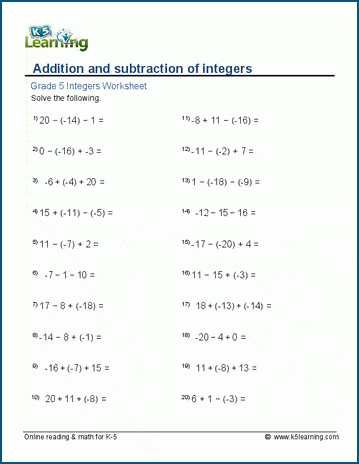## Negative numbers

Integers are whole numbers which can be greater or less than zero. Our grade 5 integer worksheets introduce concepts and operations with negative numbers, including the addition, subtraction, multiplication and division of integers.

 Absolute values | -5 | = Comparing integers -21 < -7 Integers and number lines Label -9, 12 Addition of integers 8 + (-4) = Subtraction of integers 8 - (-4) = Add & subtract integers -17 - (-8) + 12 = Multiplication of integers -6 x 11 = Division of integers -54 ÷ (-9) =